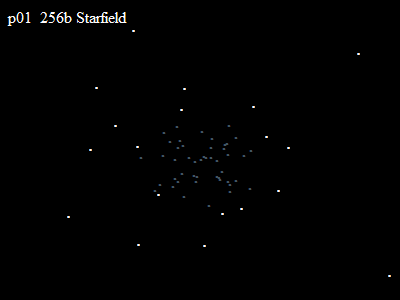# STARFIELD

109 wordsMathieu 'p01' Henri on February 25th, 2007

# Starfield

A simple 3D starfield with title and fog in 256 bytes of JavascriptThere is also a plain version in 209 bytes

## Source code

``<body id=B text=snow bgColor=0><script>O=setInterval("m='p01 256b Starfield';c=Math.cos;for(o=0;o<65;)m+='<p style=position:absolute;top:'+(50+(z=399/(73-(++o+O++&63)))*c(o*.9))+'%;left:'+(50+z*c(o))+(z>>4?'%>.':'%;color:#456>.');B.innerHTML=m",9)</script>``

256 bytes for the polished version above with title, colors and fog and 209 bytes for the plain version below

``<body id=B text=tan bgColor=0><script>setInterval("m='';c=Math.cos;for(u=65;u;)m+='<p style=position:absolute;top:'+(50+(z=399/(9+(--U+u--&63)))*c(u*9))+'%;left:'+(50+z*c(u))+'%>�';B.innerHTML=m",U=9)</script>``

The cool tricks were the TOTORO smiley `++o+O++` that increases the zoom ratio and the index of the stars, and the use of `Math.cos` with fixed angles per star to give their direction.

## Feedback

The feedback for Starfield on pouet was rather nice.